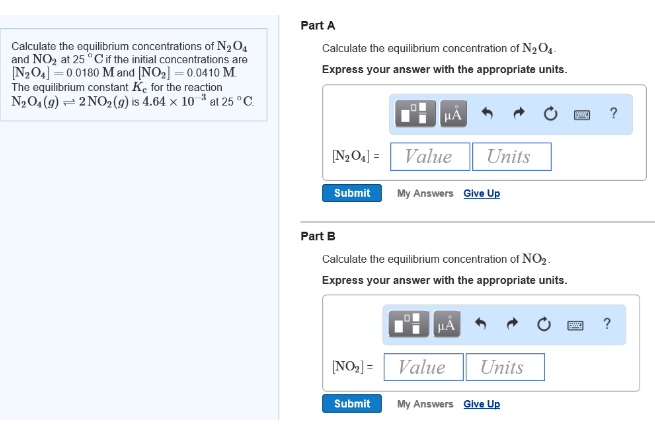# Problem: Calculate the equilibrium concentrations of N2O4 and NO2 at 25 °C if the initial concentrations are [N2O4] = 0.0180 M and [NO2] = 0.0410 M. The equilibrium constant Kc for the reaction N2O4 (g) ⇌ 2NO2 (g) is 4.64 x 10-3 at 25 °C. Part ACalculate the equilibrium concentration of N2O4. Express your answer with the appropriate units. Part BCalculate the equilibrium concentration of NO2. Express your answer with the appropriate units.

###### FREE Expert Solution
90% (285 ratings)###### Problem Details

Calculate the equilibrium concentrations of N2O4 and NO2 at 25 °C if the initial concentrations are [N2O4] = 0.0180 M and [NO2] = 0.0410 M. The equilibrium constant Kfor the reaction N2O4 (g) ⇌ 2NO(g) is 4.64 x 10-3 at 25 °C.

Part A

Calculate the equilibrium concentration of N2O4. Express your answer with the appropriate units.

Part B

Calculate the equilibrium concentration of NO2. Express your answer with the appropriate units.